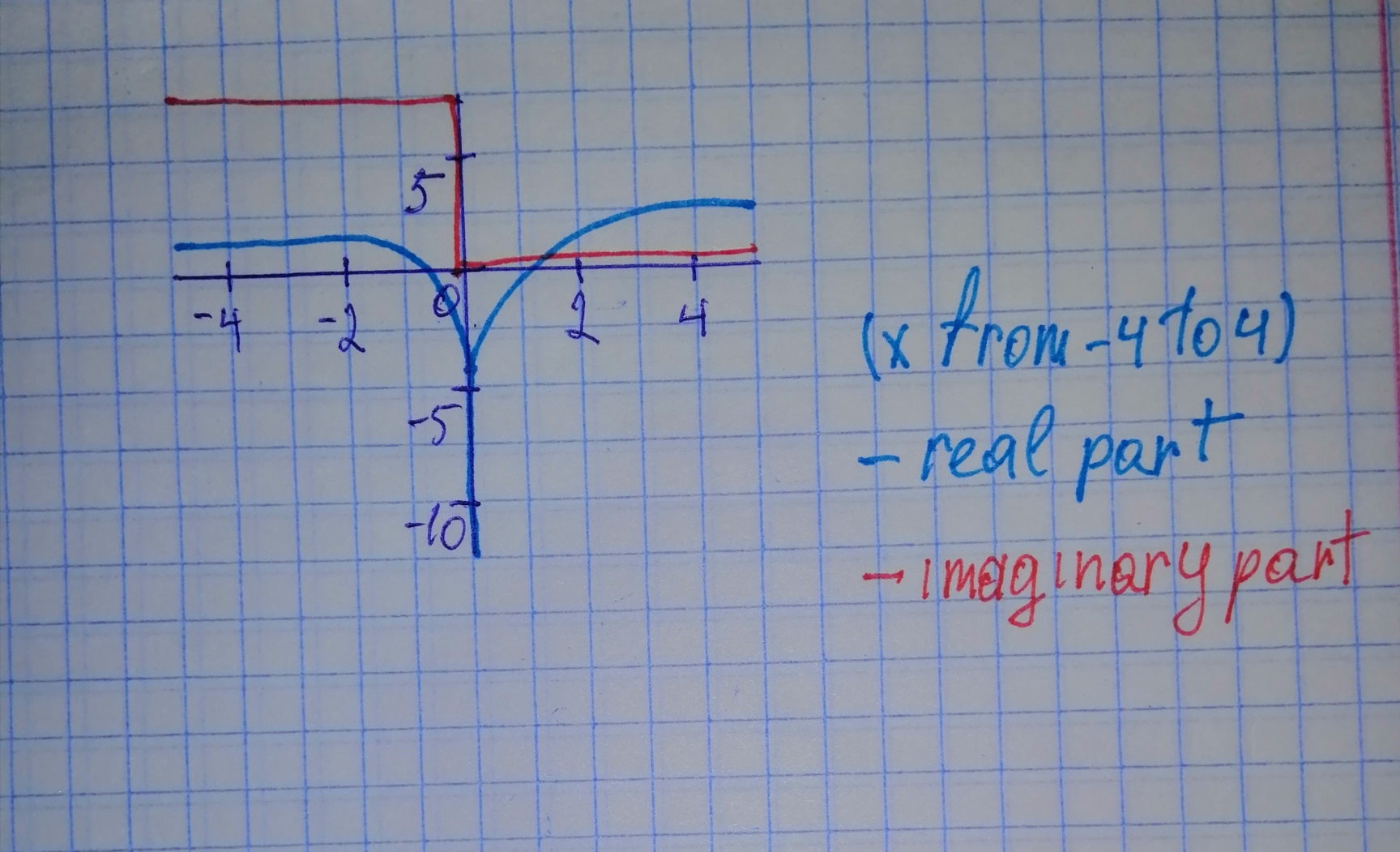# Begin by graphing f(x)=log_{2}x Then use transformations of this graph to graph the given function. What is the graph's x-intercept? What is the vertical asymptote? Use the graphs to determine each function's domain and range. r(x)=log_{2}(-x)banganX 2020-10-27 Answered
Begin by graphing $f\left(x\right)={\mathrm{log}}_{2}x$ Then use transformations of this graph to graph the given function. What is the graphs
You can still ask an expert for help

• Questions are typically answered in as fast as 30 minutes

Solve your problem for the price of one coffee

• Math expert for every subject
• Pay only if we can solve itDelorenzoz

Step 1

in the y-axis.